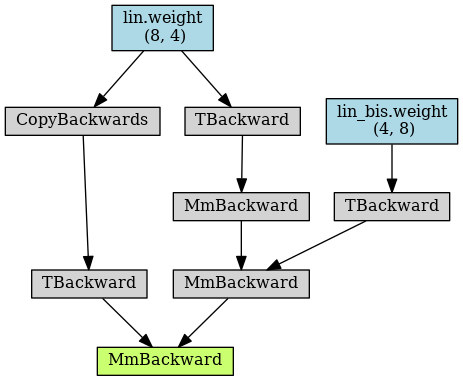# `copy_` operations get repeated in autograd computation graph

Hello,

In part of my model, a tensor that is not parameters gets modified during the `forward()` call and I want to remember it. As I use DataParallel, this tensor is registered as a buffer so that it will be saved from the first replica, as explained here in the second warning: DataParallel — PyTorch 1.8.1 documentation

The modification to this tensor needs to be in-place. Hence, I want to use `copy_`.

This is from there that I found a weird behavior for `copy_`, so I put the code below just as a sample displaying it (happening without DataParallel).

Here is my sample code:

``````import torch
import torch.nn as nn
import torch.optim as optim

from torchviz import make_dot

class MyModel(nn.Module):
def __init__(self):
super(MyModel, self).__init__()

self.lin = nn.Linear(4, 8, bias=False)

self.lin_bis = nn.Linear(8, 4, bias=False)

self.register_buffer('tensor_bidon', torch.ones(self.lin.weight.shape))

def forward(self, x):
self.tensor_bidon.copy_(self.lin.weight)

x = self.lin(x)
x = self.lin_bis(x)

x = torch.matmul(x, self.tensor_bidon.t())

return x

mymod = MyModel()

loss_mine = torch.nn.MSELoss()

mymod.train()

for i in range(5):
print(f'-------- {i} ---------')
inp = torch.randn(10, 4)
target = torch.randn(10, 8)

outputs_full = mymod(inp)

loss = loss_mine(outputs_full, target)

dot = make_dot(outputs_full, params={**{'inputs': inp}, **dict(mymod.named_parameters())})
dot.render(f"MYDIAG_SINGLE{i}", format='png')

print(mymod.lin.weight)
loss.backward()

optimizer_mine.step()
``````

Here is the first autograd graph forward (as saved with `make_dot` in my code above):As you can see (in the posts below), the CopyBackwards operation gets duplicated in the following iterations.

Do you have any hint as to why this happens?

Many thanks!Ideally, we would not want to have those duplicates CopyBackwards.

Hi,

The problem is that because you copy something the requires grad into this, that Tensor starts requirering gradients, and so you start tracking all operations that happen to it.

In general, I would recommend to just have it being a temporary variable in the forward so that you don’t need to worry about that.
If for some reason, you want to keep it on `self.`, you can detach it from the previous iterations by doing `self.tensor_bidon = self.tensor_bidon.detach()`.

Thanks a lot @albanD . That is what I ended up doing indeed, avoid the `copy_` for tensors in the gradient computation graph, and using it only in an additional buffer to save the tensor I need, where we use `copy_(whatever_tensor.detach())` (because of DataParallel requirements).